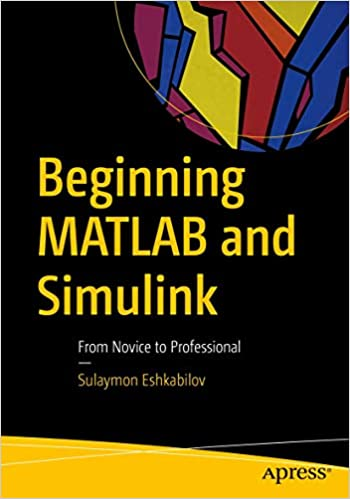# Beginning MATLAB and Simulink; From Novice to Professional

#### Category: Technical

Posted on 2020-06-16, by phaelx.

DescriptionDate: 2019
Pages: 524
ISBN10: 1484250605
ISBN13: 978-1484250600
Author: Sulaymon Eshkabilov

Size: 25 MB
Format: pdf
Employ essential and hands-on tools and functions of the MATLAB and Simulink packages, which are explained and demonstrated via interactive examples and case studies. This book contains dozens of simulation models and solved problems via m-files/scripts and Simulink models which help you to learn programming and modeling essentials. You'll become efficient with many of the built-in tools and functions of MATLAB/Simulink while solving engineering and scientific computing problems.  Beginning MATLAB and Simulink explains various practical issues of programming and modelling in parallel by comparing MATLAB and Simulink. After reading and using this book, you'll be proficient at using MATLAB and applying the source code from the book's examples as templates for your own projects in data science or engineering.   What You Will Learn*Get started using MATLAB and Simulink *Carry out data visualization with MATLAB *Gain the programming and modeling essentials of MATLAB *Build a GUI with MATLAB *Work with integration and numerical root finding methods *Apply MATLAB to differential equations-based models and simulations *Use MATLAB for data science projects Who This Book Is For Engineers, programmers, data scientists, and students majoring in engineering and scientific computing.
Software Programming Compilers,Programming Algorithms,Mathematical Logic

7523 dl's @ 2451 KB/s
6848 dl's @ 3610 KB/s
5646 dl's @ 2865 KB/s

Search More...
Beginning MATLAB and Simulink; From Novice to ProfessionalRelated Books